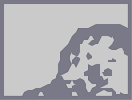### Teh Humble ZebraHover over the thumbnail for a full-size version.

Author BE_nSPIRED author:be_nspired humble n-art nonautogenerated oh-bum-nan rated zebra 2008-12-27 2008-12-27 5 by 103 people. \$Teh Humble Zebra#BE_nSPIRED#none#00000000000000000000000000000000000000000000000000000000000000000000000000000000000000000000000000000000000000000000000000000000000000000000000000000000000000000000000000000000000000000000000000000000000000000000000000000000000000000000000000000000000000000000000000000000000000000000000000000000000000000000000000000000000000000000000000000000000000000000000000000000000000000000000000000000000000000000000000000000000000000000000000000000000000000000000000000000000000000000000000000000000000000000000000000000000000000000000000000000000000000000000000000000000000000000000000000000000000000000000000000000000000000000000000000000000000000000000000000000000000000000000000000000000000000000000000000000000000000|10^599,388!10^599,384!10^601,380!10^603,374!10^605,367!10^605,357!10^607,358!10^607,350!10^607,344!10^607,337!10^607,329!10^607,327!10^605,324!10^605,320!10^605,320!10^609,323!10^613,325!10^616,327!10^620,329!10^622,330!10^627,333!10^630,337!10^630,342!10^630,346!10^630,350!10^628,357!10^624,361!10^624,361!10^620,365!10^620,367!10^620,369!10^620,374!10^620,376!10^620,379!10^616,383!10^613,387!10^613,392!10^613,396!10^616,387!10^616,388!10^605,397!10^605,392!10^605,391!10^606,388!10^608,385!10^609,384!10^610,378!10^610,377!10^610,373!10^611,365!10^612,362!10^617,355!10^618,355!10^622,351!10^622,347!10^622,345!10^623,336!10^620,335!10^618,335!10^615,339!10^615,347!10^615,351!10^613,340!10^611,346!10^611,355!10^610,371!10^606,404!10^609,408!10^609,411!10^609,417!10^609,421!10^610,423!10^610,426!10^611,430!10^611,432!10^611,436!10^611,440!10^611,442!10^613,445!10^613,449!10^613,453!10^613,456!10^613,458!10^613,465!10^613,471!10^613,476!10^613,481!10^613,482!10^612,490!10^612,491!10^613,493!10^614,495!10^614,500!10^616,503!10^616,506!10^616,510!10^616,520!10^616,522!10^616,530!10^616,533!10^616,543!10^616,545!10^617,556!10^617,570!10^610,575!10^613,575!10^616,575!10^616,572!10^616,569!10^618,563!10^618,556!10^618,551!10^618,542!10^618,540!10^618,531!10^619,518!10^619,507!10^619,499!10^621,491!10^621,483!10^621,480!10^621,478!10^621,474!10^621,473!10^621,467!10^619,461!10^619,459!10^619,455!10^619,450!10^619,443!10^619,440!10^617,434!10^617,432!10^615,430!10^615,428!10^615,425!10^615,420!10^615,416!10^615,414!10^615,411!10^615,410!10^616,394!10^617,392!10^618,399!10^616,404!10^642,459!10^642,459!10^643,451!10^643,449!10^644,445!10^645,443!10^647,441!10^649,439!10^649,435!10^650,433!10^652,431!10^654,427!10^657,420!10^658,417!10^659,415!10^661,411!10^665,400!10^673,379!10^671,379!10^668,381!10^668,383!10^668,385!10^668,389!10^666,391!10^664,393!10^664,402!10^664,402!10^641,469!10^643,464!10^645,459!10^647,456!10^649,453!10^651,449!10^653,447!10^655,445!10^657,441!10^661,436!10^663,433!10^663,432!10^666,428!10^668,425!10^669,422!10^671,420!10^673,414!10^673,412!10^675,408!10^676,404!10^676,403!10^676,399!10^676,396!10^676,394!10^676,391!10^676,388!10^672,395!10^672,404!10^667,411!10^666,412!10^672,373!10^641,548!10^645,543!10^647,540!10^649,535!10^653,529!10^657,529!10^659,525!10^662,522!10^665,518!10^668,516!10^671,514!10^675,511!10^678,503!10^679,503!10^683,499!10^685,497!10^687,496!10^689,492!10^691,489!10^693,487!10^694,483!10^697,478!10^698,474!10^701,470!10^703,465!10^705,461!10^707,458!10^709,454!10^663,515!10^664,509!10^667,503!10^669,501!10^672,496!10^675,491!10^678,489!10^682,487!10^684,485!10^686,481!10^691,477!10^694,475!10^573,303!10^570,304!10^570,305!10^567,308!10^568,312!10^568,314!10^570,316!10^572,318!10^572,320!10^572,323!10^572,323!10^570,326!10^570,327!10^570,328!10^570,330!10^570,334!10^570,335!10^568,339!10^566,340!10^566,341!10^563,344!10^563,345!10^563,348!10^560,349!10^560,354!10^560,362!10^558,357!10^558,357!10^559,367!10^560,368!10^561,372!10^561,373!10^561,376!10^563,379!10^564,380!10^565,381!10^565,384!10^567,387!10^567,388!10^567,391!10^568,393!10^568,394!10^569,397!10^569,398!10^569,401!10^569,402!10^569,403!10^569,406!10^570,407!10^572,410!10^572,410!10^572,411!10^572,416!10^572,418!10^573,420!10^573,421!10^574,423!10^575,425!10^575,426!10^575,429!10^575,430!10^575,434!10^575,435!10^575,440!10^577,443!10^577,444!10^577,447!10^577,450!10^578,451!10^578,452!10^578,453!10^578,454!10^578,455!10^578,460!10^578,463!10^578,465!10^579,467!10^579,468!10^580,470!10^580,473!10^580,476!10^580,478!10^580,479!10^580,484!10^580,484!10^581,488!10^583,495!10^583,495!10^583,497!10^583,498!10^583,499!10^584,500!10^584,507!10^585,509!10^587,511!10^589,515!10^589,516!10^589,520!10^589,523!10^589,525!10^591,530!10^591,531!10^591,532!10^591,535!10^591,538!10^591,540!10^592,544!10^593,546!10^593,549!10^593,550!10^593,553!10^595,554!10^595,555!10^595,562!10^595,566!10^595,572!10^595,574!10^595,583!10^573,438!10^570,437!10^570,435!10^570,432!10^568,427!10^566,425!10^566,422!10^566,417!10^566,414!10^566,414!10^562,411!10^562,407!10^562,403!10^562,403!10^558,400!10^558,397!10^558,395!10^558,392!10^556,386!10^554,384!10^554,381!10^554,378!10^554,376!10^551,371!10^551,369!10^549,367!10^547,363!10^543,357!10^541,356!10^539,353!10^539,350!10^539,347!10^542,343!10^542,343!10^546,341!10^550,337!10^551,334!10^549,331!10^546,327!10^546,327!10^546,327!10^549,327!10^553,323!10^556,317!10^556,315!10^552,309!10^552,308!10^555,306!10^558,306!10^564,304!10^564,304!10^564,306!10^562,313!10^563,317!10^563,320!10^563,324!10^567,331!10^557,329!10^559,329!10^560,333!10^554,344!10^552,348!10^547,360!10^548,350!10^550,348!10^554,352!10^554,356!10^547,355!10^548,346!10^552,340!10^556,340!10^560,337!10^560,336!10^555,331!10^555,326!10^559,323!10^559,321!10^557,316!10^557,316!10^666,579!10^666,577!10^670,575!10^672,573!10^674,568!10^675,566!10^678,564!10^679,564!10^682,562!10^687,560!10^689,555!10^691,553!10^693,551!10^696,547!10^701,541!10^703,534!10^697,543!10^550,305!10^548,305!10^544,306!10^541,307!10^539,307!10^536,307!10^532,307!10^529,308!10^526,311!10^522,312!10^520,314!10^520,315!10^520,317!10^516,317!10^513,319!10^511,321!10^511,325!10^512,329!10^513,333!10^510,334!10^510,337!10^510,339!10^508,343!10^508,345!10^509,352!10^511,354!10^512,359!10^513,360!10^514,362!10^516,365!10^516,366!10^518,368!10^520,370!10^521,372!10^521,378!10^522,379!10^523,384!10^524,385!10^526,388!10^528,391!10^530,395!10^531,398!10^532,400!10^534,402!10^536,407!10^536,411!10^536,415!10^540,416!10^542,418!10^542,420!10^546,424!10^548,426!10^552,429!10^552,433!10^552,437!10^554,440!10^555,444!10^556,446!10^503,320!10^503,320!10^502,320!10^500,321!10^507,319!10^510,319!10^503,330!10^505,330!10^503,334!10^501,337!10^501,339!10^501,343!10^502,348!10^505,351!10^519,379!10^515,376!10^512,376!10^510,372!10^508,372!10^569,576!10^566,574!10^566,573!10^566,573!10^567,572!10^567,569!10^567,569!10^567,566!10^567,565!10^567,562!10^569,556!10^569,556!10^569,556!10^569,554!10^569,552!10^569,549!10^569,546!10^569,545!10^570,539!10^570,538!10^570,536!10^570,533!10^570,532!10^570,530!10^570,528!10^567,524!10^567,523!10^567,521!10^567,521!10^565,519!10^565,515!10^563,511!10^563,507!10^563,503!10^563,502!10^564,531!10^562,499!10^559,495!10^559,493!10^557,491!10^557,488!10^557,487!10^557,485!10^557,485!10^555,482!10^555,479!10^553,479!10^553,476!10^553,475!10^553,472!10^551,469!10^551,469!10^551,467!10^547,465!10^547,463!10^547,461!10^547,460!10^545,458!10^543,456!10^541,454!10^539,449!10^539,449!10^539,447!10^536,444!10^536,442!10^536,439!10^534,438!10^530,433!10^528,431!10^528,429!10^526,428!10^526,426!10^524,423!10^522,422!10^522,420!10^520,418!10^520,416!10^518,415!10^516,412!10^516,409!10^516,408!10^512,404!10^512,404!10^538,582!10^540,577!10^540,577!10^541,574!10^543,569!10^543,568!10^544,566!10^545,561!10^545,558!10^545,556!10^545,553!10^545,550!10^545,548!10^545,543!10^545,541!10^545,536!10^545,534!10^545,531!10^545,528!10^547,524!10^547,523!10^545,519!10^544,515!10^544,511!10^544,510!10^544,509!10^544,507!10^541,501!10^541,501!10^541,496!10^541,495!10^538,490!10^538,489!10^534,568!10^536,567!10^536,563!10^536,561!10^536,557!10^538,550!10^538,549!10^539,545!10^540,540!10^540,537!10^540,536!10^542,530!10^542,529!10^542,528!10^542,527!10^533,575!3^587,399!3^587,402!3^588,404!3^588,405!3^589,407!3^589,411!3^589,413!3^589,418!3^590,419!3^591,423!3^591,424!3^591,428!3^592,431!3^593,438!3^593,440!3^593,445!3^593,447!3^593,449!3^593,455!3^594,459!3^595,462!3^595,465!3^595,471!3^595,476!3^597,482!3^597,486!3^598,489!3^599,491!3^599,493!3^599,503!3^599,504!3^600,506!3^601,510!3^601,513!3^602,517!3^603,518!3^596,477!3^629,434!3^629,434!3^630,429!3^631,425!3^632,422!3^633,420!3^634,415!3^636,411!3^636,409!3^636,405!3^638,403!3^640,396!3^640,394!3^642,389!3^644,383!3^647,508!3^647,506!3^648,503!3^649,500!3^650,496!3^653,492!3^654,489!3^656,488!3^658,483!3^658,482!3^662,476!3^664,476!3^666,473!3^669,471!3^671,469!3^674,465!3^677,459!3^679,456!3^682,452!3^687,445!3^688,443!3^691,437!3^692,434!3^695,427!3^697,422!3^698,418!3^652,559!3^654,559!3^656,555!3^657,553!3^658,551!3^662,549!3^664,549!3^668,543!3^670,542!3^671,540!3^585,376!3^586,388!3^677,536!3^681,533!3^682,533!3^688,525!3^694,518!3^701,511!10^411,417!10^411,419!10^413,420!10^415,423!10^416,424!10^416,426!10^411,427!10^410,428!10^410,429!10^407,429!10^403,429!10^403,429!10^400,430!10^397,431!10^394,431!10^393,431!10^389,432!10^385,433!10^383,431!10^380,431!10^379,431!10^377,433!10^371,431!10^369,431!10^366,431!10^360,431!10^359,428!10^355,425!10^352,421!10^357,422!10^359,423!10^363,425!10^366,426!10^370,426!10^376,426!10^380,426!10^385,426!10^387,426!10^390,422!10^394,420!10^395,420!10^408,415!10^400,418!10^400,420!10^399,421!10^356,435!10^356,437!10^356,439!10^356,441!10^357,444!10^359,446!10^338,407!10^335,407!10^335,403!10^335,400!10^331,397!10^329,392!10^329,389!10^330,387!10^333,384!10^335,382!10^337,382!10^341,382!10^346,382!10^348,380!10^352,378!10^357,374!10^361,372!10^368,368!10^370,368!10^375,365!10^375,365!10^378,365!10^384,365!10^386,365!10^389,363!10^389,361!10^392,361!10^395,361!10^398,361!10^400,362!10^337,387!10^340,387!10^342,387!10^345,389!10^351,389!10^354,389!10^356,387!10^357,387!10^358,387!10^360,385!10^363,386!10^366,386!10^368,386!10^372,386!10^375,384!10^377,384!10^377,384!10^382,382!10^382,382!10^385,378!10^386,376!10^382,374!10^376,374!10^373,376!10^368,377!10^363,377!10^360,377!10^356,377!10^388,380!10^391,381!10^393,381!10^395,381!10^399,381!10^402,381!10^404,381!10^407,381!10^410,383!10^412,385!10^413,387!10^415,388!10^413,384!10^413,380!10^409,376!10^405,371!10^405,368!10^402,367!10^396,368!10^396,370!10^401,374!10^398,374!10^393,371!10^334,416!10^334,421!10^334,419!10^327,412!10^326,410!10^323,408!10^320,406!10^317,404!10^317,403!10^314,400!10^314,398!10^310,396!10^310,391!10^310,389!10^310,385!10^308,382!10^308,382!10^307,379!10^307,376!10^307,371!10^307,369!10^304,363!10^304,362!10^304,354!10^304,352!10^305,348!10^306,346!10^307,344!10^309,344!10^311,344!10^313,344!10^325,342!10^325,342!10^327,340!10^328,340!10^333,340!10^336,338!10^338,338!10^339,336!10^342,336!10^344,336!10^349,334!10^355,331!10^355,331!10^319,341!10^312,341!10^309,339!10^309,338!10^309,336!10^309,335!10^312,331!10^313,330!10^316,330!10^317,330!10^321,326!10^324,326!10^323,322!10^324,323!10^329,324!10^331,325!10^331,325!10^326,329!10^326,330!10^333,331!10^339,331!10^345,329!10^343,325!10^337,323!10^343,323!10^354,323!10^354,323!10^357,323!10^358,323!10^350,325!10^346,327!10^364,328!10^364,329!10^365,330!10^367,330!10^371,330!10^374,330!10^381,330!10^383,330!10^387,330!10^391,330!10^393,332!10^394,332!10^396,333!10^397,334!10^399,336!10^401,337!10^403,339!10^396,330!10^392,326!10^388,324!10^386,322!10^377,320!10^376,320!10^374,320!10^371,320!10^367,321!10^363,321!10^362,321!10^408,346!10^375,293!10^375,293!10^370,291!10^368,289!10^365,290!10^365,292!10^360,293!10^357,294!10^354,294!10^349,292!10^348,292!10^345,292!10^340,292!10^337,292!10^336,292!10^326,287!10^330,291!10^330,289!10^327,289!10^324,287!10^323,287!10^321,288!10^318,289!10^316,289!10^314,289!10^314,289!10^312,289!10^309,289!10^306,291!10^301,291!10^300,291!10^298,291!10^294,289!10^294,289!10^293,289!10^293,285!10^293,284!10^293,283!10^297,280!10^305,280!10^308,280!10^309,280!10^310,280!10^315,278!10^317,278!10^322,278!10^326,280!10^329,281!10^332,283!10^341,285!10^349,286!10^353,286!10^361,284!10^354,281!10^349,281!10^344,279!10^340,277!10^334,275!10^332,275!10^329,273!10^325,271!10^324,269!10^322,268!10^319,268!10^319,270!10^316,271!10^313,272!10^310,273!10^306,274!10^303,276!10^303,276!10^298,277!10^294,278!10^289,281!10^287,285!10^287,288!10^287,289!10^287,295!10^287,297!10^287,299!10^288,304!10^288,308!10^288,309!10^285,310!10^285,313!10^286,316!10^286,319!10^286,323!10^286,327!10^286,330!10^286,336!10^286,341!10^286,343!10^286,345!10^286,348!10^286,350!10^286,352!10^286,356!10^286,359!10^288,362!10^288,362!10^288,364!10^288,367!10^288,373!10^289,377!10^290,379!10^290,381!10^290,382!10^290,389!10^290,391!10^290,395!10^293,403!10^294,407!10^296,408!10^298,411!10^418,350!10^418,355!10^419,356!10^420,365!10^421,366!10^422,367!10^425,371!10^425,374!10^425,379!10^426,380!10^426,384!10^426,393!10^426,393!10^426,397!10^426,401!10^426,401!10^428,404!10^429,406!10^429,410!10^430,414!10^431,419!10^431,422!10^431,425!10^431,429!10^431,435!10^431,437!10^431,440!10^431,441!10^431,444!10^431,450!10^431,454!10^429,455!10^429,458!10^429,458!10^427,462!10^425,463!10^425,468!10^422,472!10^420,473!10^416,473!10^415,474!10^410,475!10^405,475!10^401,473!10^400,471!10^393,468!10^392,468!10^390,468!10^386,465!10^382,465!10^376,461!10^376,459!10^387,475!10^389,476!10^391,479!10^393,481!10^396,485!10^399,486!10^402,490!10^405,494!10^406,504!10^407,507!10^409,514!10^410,517!10^406,499!10^413,524!10^413,525!10^413,528!10^416,532!10^417,535!10^421,538!10^424,541!10^427,544!10^429,545!10^430,547!10^433,550!10^436,552!10^440,554!10^443,555!10^446,555!10^449,555!10^451,556!10^455,556!10^460,553!10^463,553!10^466,553!10^474,553!10^476,551!10^478,549!10^479,549!10^487,544!10^488,545!10^495,539!10^497,537!10^501,532!10^503,530!10^507,524!10^509,521!10^512,516!10^519,506!10^519,506!10^521,503!10^525,496!10^527,492!10^530,485!10^514,508!10^529,484!10^529,484!10^530,482!10^533,478!10^534,474!10^534,470!10^534,468!10^534,462!10^534,458!10^534,455!10^534,455!10^534,453!10^532,450!10^532,447!10^530,443!10^526,437!10^524,431!10^520,425!10^517,419!10^517,416!10^513,409!10^509,400!10^509,400!10^509,398!10^505,394!10^505,393!10^505,389!10^505,386!10^503,383!10^501,375!10^501,373!10^501,370!10^497,364!10^495,363!10^494,361!10^491,355!10^488,351!10^485,347!10^485,347!10^483,348!10^480,348!10^471,348!10^468,348!10^465,346!10^457,340!10^456,340!10^452,341!10^443,345!10^438,346!10^426,348!10^424,350!10^430,351!10^435,347!10^440,347!10^445,345!10^459,341!10^460,341!10^469,506!10^469,506!10^467,504!10^465,504!10^465,502!10^465,502!10^463,502!10^463,502!10^463,499!10^463,498!10^460,498!10^460,496!10^460,494!10^458,492!10^456,490!10^456,489!10^454,487!10^454,485!10^452,483!10^452,480!10^452,478!10^452,478!10^452,477!10^452,474!3^436,494!3^438,499!3^441,502!3^443,504!3^447,508!10^432,360!10^438,354!10^444,360!10^450,354!10^450,354!10^456,354!10^462,360!10^462,360!10^474,360!10^480,360!10^486,360!10^486,366!10^486,372!10^486,372!10^468,366!10^456,366!10^438,366!10^438,366!10^438,366!10^432,372!10^432,378!10^456,378!10^486,378!10^450,360!10^444,354!10^432,360!10^432,360!10^444,360!10^438,396!10^438,402!10^450,402!10^456,396!10^456,408!10^456,408!10^468,396!10^480,396!10^480,408!10^492,396!10^492,396!10^492,384!10^492,372!10^480,396!10^480,396!10^480,396!10^468,408!10^468,408!10^444,396!10^444,408!10^444,408!10^432,420!10^444,420!10^456,420!10^468,420!10^480,408!10^492,408!10^492,408!10^492,420!10^492,420!10^504,420!10^504,396!10^504,408!10^480,420!10^480,432!10^492,432!10^516,432!10^516,432!10^504,432!10^468,432!10^468,432!10^456,432!10^444,432!10^444,444!10^456,444!10^468,444!10^468,444!10^480,444!10^492,444!10^504,444!10^516,444!10^516,456!10^516,456!10^504,456!10^492,456!10^468,456!10^468,456!10^456,456!10^444,456!10^480,456!10^467,418!10^471,410!10^479,404!10^490,409!10^493,441!10^483,441!10^472,437!10^462,435!10^450,424!10^446,414!10^451,413!10^462,403!10^476,394!10^504,382!10^500,410!10^494,417!10^495,422!10^504,427!10^484,401!10^449,376!10^446,370!10^443,387!10^438,381!10^450,383!10^471,383!10^479,383!10^478,370!10^468,373!10^464,379!10^456,384!10^458,388!10^470,386!10^492,384!10^494,386!10^494,389!10^435,394!10^487,391!10^473,451!10^459,449!10^446,450!10^439,450!10^444,441!10^450,429!10^457,430!10^480,445!10^489,446!10^500,447!10^510,443!10^519,436!10^514,445!10^490,433!10^483,426!10^483,419!10^473,430!10^413,482!10^415,491!10^416,492!10^414,496!10^414,504!10^416,510!10^420,519!10^421,522!10^423,525!10^428,531!10^432,533!10^435,536!10^442,541!10^446,542!10^451,543!10^453,544!10^460,544!10^472,544!10^468,534!10^460,534!10^457,534!10^451,531!10^447,531!10^442,527!10^437,520!10^435,515!10^433,512!10^430,509!10^430,505!10^427,502!10^424,486!10^425,484!10^440,485!10^444,493!10^455,501!10^459,508!10^457,514!10^451,529!10^443,521!10^451,516!10^446,520!10^424,497!10^459,519!10^457,521!10^466,522!10^476,510!10^480,517!10^473,519!10^476,510!10^478,502!10^478,496!10^476,493!10^471,488!10^466,482!10^464,478!10^460,470!10^454,465!10^444,471!10^439,477!10^433,478!10^430,473!10^441,458!10^440,465!10^474,462!10^475,465!10^489,464!10^510,457!10^520,455!10^532,456!10^518,465!10^515,470!10^517,474!10^481,533!10^484,537!10^475,531!10^480,521!10^485,523!10^489,525!10^497,520!10^502,512!10^505,507!10^508,503!10^511,496!10^513,489!10^516,483!10^518,474!10^467,468!10^472,471!10^478,473!10^489,473!10^498,466!10^502,461!10^505,464!10^507,469!10^499,478!10^498,484!10^495,492!10^495,509!10^490,510!10^490,508!10^488,501!10^498,497!10^503,489!10^510,474!10^488,489!10^486,485!10^475,480!10^488,480!10^525,475!10^532,464!10^527,460!10^512,541!10^512,544!10^515,546!10^515,548!10^515,551!10^515,557!10^515,558!10^513,562!10^513,564!10^513,572!10^509,574!10^498,568!10^498,567!10^498,562!10^496,557!10^484,578!10^483,578!10^479,574!10^479,571!10^479,569!10^477,566!10^476,564!10^476,562!10^474,562!10^474,565!10^474,569!10^474,573!10^472,577!10^382,294!10^384,295!10^388,299!10^392,300!10^396,302!10^400,307!10^406,312!10^410,317!10^412,322!10^416,329!10^416,335!10^419,NaN!10^286,228!10^286,229!10^287,233!10^289,236!10^290,237!10^291,241!10^293,243!10^295,244!10^297,244!10^299,244!10^301,244!10^304,244!10^309,244!10^312,240!10^313,240!10^316,238!10^318,238!10^320,238!10^324,240!10^326,241!10^330,242!10^334,245!10^338,246!10^340,249!10^345,250!10^348,253!10^350,256!10^352,258!10^356,260!10^358,260!10^360,262!10^363,264!10^365,265!10^369,266!10^372,268!10^374,270!10^376,270!10^378,273!10^387,280!10^383,278!10^387,279!10^388,283!10^394,287!10^397,290!10^399,291!10^400,295!10^401,299!10^404,303!10^348,243!10^347,243!10^347,243!10^347,238!10^347,233!10^347,229!10^343,225!10^343,222!10^343,219!10^340,215!10^340,213!10^336,207!10^332,204!10^330,200!10^329,199!10^326,197!10^323,191!10^318,189!10^315,189!10^311,189!10^307,189!10^302,190!10^295,191!10^291,191!10^298,219!10^301,220!10^303,221!10^305,222!10^308,223!10^312,223!10^315,221!10^317,219!10^321,219!10^322,219!10^326,219!10^326,219!10^328,219!10^330,219!10^330,219!10^330,216!10^299,218!10^299,215!10^297,212!10^297,209!10^297,206!10^297,203!10^299,202!10^300,200!10^301,200!10^304,200!10^310,200!10^317,200!10^321,200!10^324,203!10^326,206!10^329,208!10^326,210!10^323,213!10^317,215!10^314,217!10^309,217!10^306,213!10^367,132!10^367,136!10^367,139!10^364,143!10^364,147!10^362,150!10^362,153!10^360,157!10^360,160!10^360,163!10^360,166!10^360,168!10^360,172!10^360,175!10^360,179!10^358,182!10^356,183!10^356,187!10^357,190!10^359,195!10^361,197!10^361,199!10^363,204!10^364,206!10^365,208!10^369,212!10^373,216!10^376,217!10^380,221!10^381,226!10^385,229!10^389,232!10^391,235!10^396,243!10^397,245!10^399,247!10^403,249!10^403,253!10^404,256!10^406,260!10^407,264!10^408,267!10^411,270!10^413,272!10^413,276!10^414,279!10^415,284!10^417,285!10^417,285!10^419,287!10^421,289!10^422,291!10^423,295!10^425,298!10^425,300!10^427,302!10^431,308!10^431,310!10^431,310!10^433,314!10^435,317!10^437,319!10^439,323!10^441,324!10^441,335!10^441,335!10^443,328!10^360,224!10^361,229!10^364,232!10^366,235!10^370,240!10^371,242!10^373,244!10^378,248!10^380,250!10^382,252!10^384,253!10^386,256!10^388,259!10^392,263!10^394,266!10^395,268!10^396,271!10^378,146!10^376,146!10^376,149!10^377,152!10^377,153!10^377,157!10^377,160!10^379,163!10^379,166!10^380,172!10^381,174!10^382,175!10^383,183!10^383,184!10^385,187!10^386,190!10^389,201!10^390,203!10^386,195!10^390,206!10^395,211!10^396,211!10^397,215!10^399,218!10^401,219!10^405,226!10^406,227!10^409,230!10^409,233!10^415,236!10^415,239!10^416,242!10^444,335!10^402,278!10^409,287!10^414,298!10^420,307!10^427,319!10^417,244!10^417,246!10^418,251!10^420,254!10^423,259!10^423,263!10^426,269!10^429,275!10^431,276!10^431,279!10^432,281!10^435,287!10^437,288!10^439,290!10^439,294!10^442,297!10^443,299!10^443,307!10^445,309!10^447,312!10^447,314!10^450,317!10^451,319!10^453,324!10^454,326!10^455,329!10^455,331!10^455,332!10^456,334!10^323,176!10^323,177!10^323,179!10^328,184!10^334,173!10^335,171!10^336,170!10^340,161!10^340,157!10^344,150!10^344,147!10^348,138!10^349,135!10^350,130!10^352,126!10^354,121!10^357,117!10^360,115!10^362,113!10^333,168!10^335,162!10^337,155!10^341,144!10^370,459!10^368,459!10^365,457!10^363,454!10^361,450!10^359,450!10^356,448!10^354,446!10^348,442!10^345,439!10^341,436!10^338,434!10^338,432!10^334,429!10^327,425!10^327,423!10^322,423!10^317,423!10^314,423!10^307,417!10^305,417!10^304,417!10^301,415!10^574,303!10^575,303!10^578,304!10^581,305!10^583,306!10^586,307!10^588,308!10^590,309!10^592,310!10^596,312!10^598,313!10^602,315!10^632,334!10^635,334!10^638,336!10^640,338!10^643,341!10^646,341!10^649,343!10^650,345!10^654,347!10^657,349!10^659,352!10^661,354!10^664,358!10^665,360!10^668,364!10^673,369!10^675,372!10^626,330!10^625,330!10^621,327!10^620,327!10^617,323!10^615,321!10^610,319!10^605,315!10^674,376!10^676,376!10^678,377!10^680,381!10^682,384!10^684,388!10^687,391!10^688,392!10^690,395!10^692,396!10^694,399!10^696,404!10^698,408!10^698,410!10^700,412!10^700,413!10^701,416!10^702,419!10^703,423!10^704,425!10^706,428!10^706,429!10^706,431!10^706,433!10^707,436!10^708,438!10^710,442!10^710,444!10^710,447!10^710,452!10^710,455!10^711,460!10^711,462!10^711,466!10^711,471!10^711,479!10^711,485!10^711,491!10^711,491!10^711,496!10^711,496!10^709,497!10^709,502!10^709,506!10^709,513!10^709,519!10^702,527!10^707,521!10^707,525!10^705,528!10^703,532!10^701,540!10^701,548!10^699,555!10^699,558!10^697,563!10^693,566!10^693,575!10^693,578!10^696,571!10^495,317!10^499,314!10^506,311!10^511,309!10^517,309!10^520,309!10^454,564!10^455,567!10^455,570!10^455,576!10^431,579!10^431,577!10^431,572!10^431,568!10^431,561!10^431,558!10^423,548!10^423,548!10^423,550!10^423,554!10^423,559!10^423,561!10^423,566!10^423,570!10^423,573!10^423,576!10^424,576!10^428,574!10^371,577!10^368,577!10^369,577!10^372,575!10^373,573!10^375,570!10^376,568!10^378,565!10^379,563!10^379,562!10^381,557!10^381,557!10^382,553!10^383,551!10^383,550!10^384,545!10^385,540!10^385,537!10^385,533!10^386,526!10^386,523!10^387,508!10^387,507!10^387,504!10^387,490!10^387,489!10^385,479!10^388,486!10^389,491!10^389,498!10^389,503!10^389,510!10^389,514!10^394,575!10^394,573!10^396,569!10^398,565!10^398,562!10^398,558!10^399,550!10^400,547!10^400,543!10^400,539!10^400,536!10^400,534!10^398,529!10^398,527!10^398,522!10^398,516!10^395,506!10^395,502!10^396,500!10^397,513!10^395,522!10^395,528!10^389,537!10^391,547!10^387,559!10^383,570!10^386,571!10^387,571!10^380,575!10^383,575!10^389,563!10^389,555!10^391,547!3^421,533!3^416,533!3^415,531!3^415,528!3^415,522!3^411,516!3^411,514!3^411,509!3^409,504!3^407,497!3^403,493!3^400,487!3^390,477!3^386,471!3^383,469!3^377,462!3^375,462!3^373,462!3^370,460!3^379,462!3^380,462!3^384,466!3^385,467!3^386,470!3^388,472!3^390,474!3^392,477!3^395,481!3^398,482!3^400,485!3^403,488!10^328,574!10^330,572!10^333,572!10^335,568!10^336,566!10^338,564!10^339,564!10^342,562!10^343,560!10^345,560!10^346,558!10^347,556!10^349,554!10^350,550!10^351,548!10^353,545!10^355,544!10^355,542!10^357,540!10^358,537!10^359,533!10^359,532!10^361,528!10^362,527!10^363,525!10^363,525!10^363,525!10^363,521!10^363,520!10^364,516!10^367,511!10^367,507!10^367,506!10^367,505!10^367,501!10^367,501!10^367,497!10^367,496!10^368,493!10^368,493!10^369,488!10^369,483!10^369,480!10^369,478!10^308,578!10^310,578!10^312,575!10^315,571!10^316,567!10^318,563!10^319,561!10^320,560!10^320,558!10^322,555!10^322,553!10^323,550!10^324,547!10^326,543!10^328,539!10^330,534!10^331,533!10^332,527!10^333,527!10^336,523!10^336,521!10^338,518!10^340,515!10^344,511!10^344,507!10^347,503!10^348,498!10^350,493!10^351,488!10^352,486!10^354,481!10^356,477!10^357,475!10^359,470!10^360,469!10^326,570!10^326,566!10^327,562!10^329,557!10^331,553!10^333,550!10^334,547!10^336,544!10^337,542!10^341,537!10^343,530!10^345,528!10^345,527!10^347,525!10^349,525!10^353,519!10^355,515!10^356,513!10^360,508!10^360,508!10^363,502!10^339,559!10^346,546!10^352,530!10^353,528!10^349,538!10^339,546!10^339,550!10^342,543!10^346,537!10^314,518!10^315,518!10^317,513!10^319,511!10^321,510!10^322,508!10^323,507!10^325,504!10^327,501!10^327,501!10^331,496!10^331,495!10^335,490!10^335,488!10^336,486!10^338,484!10^339,481!10^341,479!10^343,472!10^343,471!10^345,466!10^347,463!10^347,461!10^347,460!10^347,458!10^347,456!10^353,447!10^281,543!10^281,543!10^282,543!10^286,543!10^288,543!10^291,538!10^294,536!10^295,534!10^299,530!10^302,528!10^306,524!10^310,520!10^312,520!10^313,515!10^314,510!10^318,504!10^320,499!10^322,499!10^324,497!10^325,494!10^327,490!10^328,488!10^331,487!10^332,484!10^334,483!10^337,477!10^338,475!10^343,470!10^201,415!10^201,420!10^203,424!10^204,428!10^204,433!10^205,438!10^206,440!10^207,442!10^208,445!10^209,449!10^210,452!10^211,454!10^213,458!10^214,460!10^214,462!10^214,467!10^216,471!10^217,473!10^218,474!10^218,478!10^222,484!10^224,485!10^224,487!10^226,493!10^227,494!10^228,495!10^230,500!10^232,502!10^236,507!10^236,507!10^238,510!10^238,512!10^243,519!10^243,519!10^243,520!10^246,522!10^244,524!10^244,524!10^249,529!10^249,531!10^254,533!10^255,534!10^258,540!10^261,541!10^261,542!10^265,545!10^268,545!10^270,545!10^274,545!10^281,541!10^285,539!10^291,530!10^296,527!10^299,522!10^302,518!10^308,509!10^277,536!10^274,536!10^271,536!10^265,534!10^262,532!10^259,525!10^256,525!10^254,525!10^252,522!10^249,519!10^247,514!10^247,511!10^244,507!10^240,504!10^237,502!10^235,497!10^233,492!10^233,487!10^229,481!10^225,477!10^225,472!10^222,470!10^218,463!10^218,461!10^215,453!10^215,449!10^211,442!10^211,438!10^207,432!10^207,423!10^203,413!10^203,407!10^203,406!10^203,398!10^309,170!10^309,168!10^310,164!10^310,163!10^312,159!10^313,156!10^314,153!10^316,151!10^318,148!10^322,144!10^326,138!10^327,136!10^328,133!10^330,129!10^332,127!10^334,119!10^335,116!10^336,114!10^339,110!10^342,104!10^342,103!10^344,100!10^347,95!10^350,91!10^354,89!10^358,89!10^365,89!10^343,110!10^345,110!10^349,104!10^351,100!10^356,95!10^307,470!10^308,467!10^309,465!10^313,463!10^316,461!10^317,461!10^320,457!10^321,455!10^324,453!10^325,451!10^328,449!10^332,444!10^333,442!10^334,437!10^332,435!10^328,435!10^324,435!10^324,435!10^323,437!10^319,438!10^317,440!10^315,441!10^315,443!10^312,445!10^310,449!10^308,451!10^306,453!10^304,457!10^302,458!10^300,459!10^300,460!10^300,462!10^301,467!10^301,467!10^301,470!10^299,470!10^293,470!10^293,471!10^293,472!10^288,472!10^286,472!10^281,472!10^280,472!10^279,472!10^272,472!10^268,470!10^264,467!10^262,467!10^257,465!10^254,463!10^251,459!10^249,459!10^245,454!10^243,452!10^242,452!10^242,448!10^239,444!10^239,442!10^239,441!10^235,439!10^233,433!10^228,426!10^228,426!10^226,423!10^224,418!10^224,413!10^224,411!10^224,405!10^221,401!10^218,395!10^218,393!10^218,391!10^218,388!10^216,386!10^216,379!10^214,377!10^214,374!10^214,368!10^214,359!10^214,351!10^214,346!10^214,342!10^215,339!10^215,346!10^217,354!10^213,359!10^213,361!10^216,367!10^218,369!10^221,375!10^225,385!10^228,390!10^226,390!10^226,385!10^226,375!10^222,368!10^203,393!10^203,391!10^203,387!10^205,384!10^205,380!10^206,374!10^207,364!10^207,362!10^207,353!10^207,350!10^269,462!10^269,460!10^266,458!10^264,454!10^262,453!10^261,451!10^258,449!10^255,446!10^253,443!10^249,436!10^247,432!10^245,431!10^243,427!10^238,423!10^236,419!10^234,417!10^232,413!10^228,394!10^285,464!10^295,464!10^276,459!10^197,425!10^198,429!10^198,434!10^196,438!10^196,444!10^194,450!10^192,455!10^192,460!10^192,460!10^192,460!10^192,460!10^192,461!10^190,464!10^189,465!10^189,469!10^189,474!10^187,477!10^185,480!10^182,485!10^182,489!10^179,491!10^176,495!10^176,500!10^172,504!10^170,509!10^168,516!10^168,521!10^168,527!10^164,534!10^160,541!10^158,548!10^154,554!10^154,559!10^152,565!10^152,571!10^152,571!10^156,576!10^159,576!10^159,574!10^159,572!10^157,566!10^157,558!10^157,551!10^183,577!10^177,572!10^177,568!10^177,564!10^177,559!10^177,558!10^173,550!10^173,547!10^173,541!10^173,538!10^174,528!10^175,518!10^182,506!10^186,496!10^190,487!10^194,479!10^194,484!10^194,493!10^194,498!10^190,506!10^190,509!10^190,518!10^190,527!10^192,533!10^193,534!10^196,538!10^198,543!10^200,545!10^204,549!10^207,551!10^210,555!10^214,558!10^218,560!10^224,565!10^230,569!10^239,572!10^239,574!10^236,575!10^229,575!10^221,575!10^210,575!10^199,575!10^190,575!10^188,573!10^188,570!10^204,571!10^211,571!10^214,569!10^200,562!10^187,562!10^184,559!10^189,558!10^199,563!10^203,564!10^196,555!10^191,553!10^185,550!10^184,549!10^184,539!10^184,535!10^184,532!10^184,524!10^184,515!10^181,505!10^242,551!10^239,547!10^236,547!10^230,541!10^228,539!10^220,532!10^218,528!10^212,522!10^212,520!10^210,516!10^208,511!10^206,511!10^205,507!10^205,503!10^199,498!10^198,495!10^198,490!10^198,485!10^196,475!10^194,465!10^194,463!10^197,471!10^199,477!10^201,480!10^201,484!10^203,489!10^205,491!10^207,493!10^207,494!10^209,498!10^211,502!10^211,507!10^214,510!10^216,512!10^219,516!10^220,518!10^223,521!10^225,526!10^227,530!10^229,535!10^230,538!10^231,539!10^233,540!10^296,418!10^296,419!10^293,419!10^289,419!10^284,419!10^283,419!10^280,416!10^276,413!10^274,411!10^269,409!10^269,409!10^266,409!10^264,407!10^260,404!10^258,404!10^258,401!10^254,399!10^254,397!10^250,394!10^250,390!10^246,388!10^244,384!10^244,381!10^244,380!10^242,377!10^242,374!10^242,369!10^240,368!10^238,363!10^238,360!10^238,356!10^234,352!10^234,349!10^234,347!10^234,345!10^234,341!10^234,339!10^234,337!10^234,330!10^234,329!10^231,324!10^231,322!10^231,321!10^231,320!10^231,316!10^231,313!10^227,309!10^227,305!10^227,304!10^227,299!10^227,295!10^227,291!10^225,287!10^225,285!10^225,282!10^225,281!10^225,278!10^225,275!10^210,337!10^210,333!10^210,329!10^212,325!10^212,322!10^212,319!10^213,316!10^214,313!10^214,310!10^216,304!10^216,302!10^216,297!10^219,290!10^219,288!10^220,280!10^220,272!10^221,268!10^222,263!10^222,255!10^224,248!10^224,244!10^228,238!10^228,228!10^228,223!10^228,227!10^228,235!10^228,223!10^228,221!10^224,214!10^224,208!10^226,202!10^226,201!10^232,188!10^247,120!10^248,121!10^248,126!10^244,131!10^242,134!10^242,137!10^240,141!10^240,145!10^240,148!10^236,154!10^236,157!10^236,159!10^236,163!10^236,164!10^234,172!10^231,176!10^231,182!10^231,190!10^229,194!10^229,200!10^229,206!10^227,213!10^281,252!10^281,256!10^281,263!10^278,272!10^274,278!10^274,283!10^274,287!10^274,294!10^274,298!10^272,306!10^272,310!10^272,317!10^272,325!10^272,333!10^270,342!10^270,348!10^270,353!10^270,362!10^270,368!10^272,378!10^275,387!10^275,389!10^277,392!10^257,365!10^257,362!10^257,358!10^257,353!10^258,349!10^258,343!10^258,336!10^258,327!10^258,318!10^258,311!10^258,301!10^258,296!10^258,292!10^258,281!10^258,272!10^258,265!10^258,255!10^259,247!10^260,241!10^262,235!10^262,231!10^263,226!10^264,217!10^264,211!10^264,202!10^264,198!10^264,190!10^264,183!10^264,177!10^262,173!10^262,170!10^278,209!10^278,206!10^278,200!10^275,191!10^270,184!10^266,177!10^257,350!10^257,347!10^257,340!10^257,334!10^257,331!10^257,329!10^257,327!10^257,321!10^257,317!10^257,315!10^259,311!10^259,305!10^259,301!10^257,295!10^257,286!10^258,280!10^258,276!10^258,268!10^258,258!10^258,253!10^258,247!10^260,242!10^261,237!10^261,234!10^263,228!10^263,225!10^263,213!10^263,212!10^278,204!10^282,257!10^282,259!10^280,266!10^280,270!10^278,275!10^272,278!10^273,293!10^273,297!10^274,303!10^274,316!10^274,325!10^274,328!10^274,342!10^274,358!10^274,373!10^277,381!10^244,304!10^244,301!10^244,298!10^244,293!10^244,288!10^244,284!10^244,278!10^244,274!10^242,268!10^242,264!10^242,257!10^242,256!10^242,250!10^243,244!10^245,239!10^246,231!10^247,223!10^247,219!10^247,212!10^247,208!10^247,202!10^247,197!10^247,194!10^247,190!10^469,334!10^469,331!10^469,326!10^465,320!10^463,315!10^461,311!10^461,306!10^461,304!10^459,299!10^457,297!10^457,293!10^457,289!10^455,284!10^453,280!10^453,276!10^451,273!10^447,264!10^445,262!10^445,260!10^445,256!10^441,253!10^439,248!10^437,245!10^437,239!10^437,234!10^437,233!10^433,228!10^431,225!10^430,223!10^430,217!10^426,213!10^426,210!10^420,200!10^419,198!10^419,195!10^415,190!10^415,185!10^415,184!10^415,182!10^411,175!10^407,168!10^404,161!10^404,159!10^400,152!10^403,158!10^405,164!10^411,205!10^409,201!10^407,198!10^405,195!10^403,191!10^401,185!10^401,182!10^401,180!10^399,177!10^394,170!10^394,166!10^482,337!10^482,333!10^482,330!10^478,323!10^478,320!10^478,317!10^474,311!10^474,307!10^470,299!10^470,297!10^470,281!10^470,276!10^466,271!10^462,265!10^462,261!10^462,257!10^458,252!10^458,247!10^454,238!10^454,236!10^454,231!10^452,222!10^450,214!10^450,212!10^448,208!10^448,205!10^446,201!10^446,196!10^446,189!10^443,182!10^441,179!10^439,177!10^436,173!10^433,170!10^431,166!10^428,163!10^479,297!10^481,291!10^481,288!10^481,284!10^481,273!10^481,272!10^481,269!10^481,268!10^481,261!10^479,258!10^477,250!10^477,248!10^475,248!10^474,244!10^474,241!10^474,238!10^472,234!10^472,227!10^472,225!10^470,224!10^470,218!10^470,212!10^470,209!10^470,204!10^472,201!10^475,196!10^475,193!10^478,188!10^479,183!10^479,181!10^479,174!10^479,174!10^475,171!10^471,167!10^469,166!10^466,164!10^462,162!10^459,158!10^457,155!10^455,150!10^451,147!10^448,143!10^446,142!10^441,135!10^441,134!10^441,132!10^439,131!10^436,129!10^434,126!10^434,125!10^434,124!10^429,119!10^427,116!10^427,116!10^422,108!10^422,103!10^422,102!10^461,196!10^461,191!10^461,186!10^456,179!10^452,172!10^450,170!10^445,166!10^440,160!10^437,157!10^436,154!10^431,151!10^424,144!10^417,140!10^416,140!10^414,138!10^409,136!10^399,128!10^404,133!10^285,178!10^282,172!10^280,169!10^280,166!10^280,163!10^281,158!10^282,153!10^284,150!10^284,148!10^289,143!10^289,140!10^290,133!10^290,132!10^290,131!10^290,123!10^292,123!10^296,119!10^298,114!10^301,112!10^306,104!10^307,101!10^310,97!10^312,93!10^295,171!10^295,163!10^296,159!10^298,155!10^300,152!10^305,146!10^305,143!10^308,136!10^308,135!10^310,130!10^311,127!10^312,124!10^314,119!10^317,114!10^320,110!10^322,105!10^326,97!10^326,96!10^331,91!10^334,87!10^339,82!10^344,78!10^350,72!10^374,136!10^374,133!10^374,132!10^374,126!10^374,121!10^375,113!10^375,110!10^373,105!10^370,103!10^367,98!10^368,95!10^371,86!10^371,81!10^365,77!10^366,77!10^374,77!10^377,75!10^386,68!10^384,61!10^382,56!10^378,45!10^372,38!10^367,32!10^388,79!10^386,77!10^385,86!10^385,91!10^381,93!10^378,94!10^380,96!10^383,97!10^385,106!10^386,118!10^386,130!10^387,148!10^387,145!10^390,137!10^392,127!10^393,115!10^394,106!10^400,100!10^398,98!10^392,96!10^387,90!10^404,115!10^410,118!10^419,123!10^252,117!10^253,115!10^256,111!10^256,103!10^256,102!10^256,98!10^256,93!10^256,86!10^256,85!10^274,91!10^270,89!10^264,84!10^261,80!10^258,76!10^251,71!10^246,65!10^243,61!10^237,50!10^237,48!10^237,43!10^237,37!10^238,28!10^240,28!10^239,53!10^427,97!10^430,97!10^434,97!10^439,97!10^446,95!10^450,89!10^452,89!10^456,89!10^461,89!10^466,82!10^469,80!10^476,76!10^477,76!10^481,70!10^482,68!10^486,64!10^488,62!10^490,60!10^495,55!10^498,50!10^502,45!10^505,42!10^508,34!10^510,30!3^371,64!3^365,57!3^363,56!3^360,51!3^352,44!3^344,40!3^339,43!3^339,52!3^347,56!3^361,64!3^354,64!3^348,53!3^353,59!3^346,67!3^340,70!3^329,74!3^334,63!3^335,59!3^253,40!3^256,32!3^258,28!3^253,36!3^253,50!3^256,56!3^251,41!3^247,29!3^247,57!3^254,58!3^260,43!3^267,31!3^268,31!3^264,43!3^259,53!3^283,51!3^311,35!3^309,31!3^307,32!3^301,37!3^301,44!3^301,50!3^301,53!3^301,60!3^301,64!3^298,67!3^290,69!3^289,71!3^286,79!3^282,80!3^279,80!3^273,75!3^265,68!3^263,63!3^272,61!3^274,65!3^277,69!3^282,60!3^290,52!3^288,53!3^275,57!3^271,51!3^273,44!3^282,42!3^294,35!3^286,27!3^275,30!3^283,28!3^296,28!3^297,28!3^282,40!3^451,80!3^456,76!3^462,70!3^465,66!3^470,60!3^474,58!3^479,53!3^484,49!3^491,41!3^495,35!3^495,30!3^490,28!3^483,29!3^479,30!3^475,37!3^475,43!3^481,39!3^485,33!3^474,47!3^467,60!3^462,65!3^452,75!3^443,81!3^440,83!3^437,77!3^441,66!3^445,60!3^454,60!3^461,53!3^463,48!3^467,37!3^467,31!3^457,25!3^453,33!3^453,43!3^451,51!3^440,51!3^440,38!3^445,28!3^440,45!3^432,49!3^428,44!3^426,54!3^424,61!3^420,70!3^428,84!3^433,88!3^431,64!3^437,58!3^384,37!3^388,37!3^393,33!3^382,31!3^375,31!3^390,55!3^412,86!3^412,86!3^270,159!3^270,151!3^277,133!3^281,123!3^247,162!3^254,141!3^260,122!3^246,177!3^255,152!3^258,145!3^262,138!3^269,131!3^274,106!3^264,102!3^288,93!3^286,107!3^278,117!3^297,98!3^299,90!3^320,64!3^311,81!3^301,83!3^304,77!3^317,76!3^313,54!3^322,44!3^333,30!3^345,33!3^319,30!3^358,32!3^406,87!3^399,73!3^409,33!3^407,55!3^244,482!3^254,501!3^276,523!3^287,517!3^294,515!3^258,506!3^265,514!3^242,483!3^235,470!3^255,428!3^268,441!3^276,449!3^289,454!3^298,455!3^207,529!3^218,551!3^224,556!3^232,561!3^242,566!3^255,569!3^266,575!3^246,421!3^225,463!3^242,495!3^578,315!3^587,318!3^601,334!3^535,319!3^524,323!3^540,314!3^638,351!3^646,357!3^654,363!3^658,367!3^688,410!3^688,421!3^702,439!3^703,445!3^633,580!3^636,571!3^639,562!3^525,520!3^528,514!3^560,460!3^564,479!3^328,314!3^333,314!3^345,314!3^368,312!3^349,372!3^362,366!3^373,360!3^379,358!3^370,421!3^376,422!3^391,420!3^475,480!3^476,451!3^474,439!3^474,424!3^463,412!3^463,414!3^463,434!3^471,447!3^477,455!3^486,455!3^491,449!3^489,438!3^486,418!3^480,406!3^469,384!3^474,208!3^480,203!3^484,189!3^482,197!3^263,86!3^263,84!3^257,78!3^254,76!3^252,75!3^248,71!3^245,66!3^243,64!3^241,59!3^239,55!3^237,51!3^237,47!3^237,42!3^237,37!3^237,33!3^237,29!3^439,97!3^442,97!3^445,95!3^450,89!3^454,85!3^460,85!3^463,85!3^468,79!3^471,75!3^473,73!3^479,71!3^481,68!3^484,64!3^487,60!3^490,59!3^496,53!3^500,48!3^502,44!3^504,41!3^505,38!3^508,33!3^508,32!3^508,27!10^388,81!10^388,78!10^388,74!10^389,68!10^390,66!10^388,61!10^388,55!10^385,53!10^380,49!10^376,41!10^372,36!10^375,41!10^376,49!10^378,57!10^379,64!10^296,62!10^299,61!10^299,59!10^301,56!10^302,47!10^302,45!10^300,39!10^297,34!10^280,79!10^287,79!10^290,73!10^294,69!10^298,63!10^298,59!10^302,50!10^302,41!10^302,36!10^300,32!10^297,29!10^299,38!10^283,83!10^464,30!10^458,32!10^454,33!10^450,38!10^447,42!10^442,46!10^432,49!10^429,52!10^429,54!10^434,46!10^444,37!10^440,91!10^435,91!10^430,87!10^427,84!10^431,86!10^436,87!10^444,42!3^377,414!3^389,414!3^411,461!3^419,455!3^421,446# I said on 'Teh Bengal TIger' map that i had another n-art in the making, i would say it took about 8 hours in total also spread out like the other, this time i have removed my signature and will be happy to take advice on my next n-art, ooh i wonder how this will go down to n-arts by the same person on the hotmaps, i dont think thats happened before, at least not since ive been around

## Other maps by this authorbottom Left bottom right Top Left Mirror's Edge Chinese Dragon Tileset Teh Bengal Tiger

Pages: (2) [ 1 ] 2

### Cool

I was the 100th rater on this map!
first for me.

### amazing!!!

sorry didnt see this before or you as an author im kinda newish 5/5

cool :)
5aved

### very nice ^^

really like it a lot =]

5/5.
wow [nmaps.net]

### i think

u can agree with my latest comment on zivilynbanes map

and i thought i rated this guess not

### I cant believe I missed this!

I like all but the nose... good work... your art keeps getting better.

### you are realy good!

you are the best in N art that I have EVER seen!

### Good riddance.

Nice map though.
JUST GOT KICKED OF THE FIRST ON HOT MAPS
34 hours on top and moves down to second place on hot maps page.
5

### haha!

comment 87!!
finally.....
stop rate this map please.... this is outrageous how long time its been on top of hotpage

5aved

### O__o

It's like this map is glued to the top of this page!

### 3/5

i dont like n arts with only one kind of objects
and and a zebra doesnt seem that exciting for me...

### It's very well done

and must have taken a lot of effort, but the image really isn't very interesting for me.

3/5 - the ability to produce a good Nart is as much to do with choosing a subject as it is producing the work (imo).

### Holy Crap.

30 hours and still on the top of the hot maps page. 5/5

### This is why I faved it

I was showing my appreciation for the N-art without having to give it a rating that kept it on the Hot Maps page.

It's not that I hate N-arts (as crescor claimed in an earlier comment) but that I hate how there seems to be little to no check on comparing n-arts to each other. We do it all the time with action, puzzle, race maps alike without even noticing it. Every map has to be as enjoyable as another map. When I play an amazing map I notice that I rate maps a little less than I probably would have if I had not played such a great map earlier.

N-arts seem to just garner high and low votes as people try to knock them off the Hot Maps page but also try to show their support for the art in general.

Faving the thing gets around this problem. I didn't add to the map staying on the Hot Maps page, but I still showed that I support the drawing that BE_nSPIRED has made. Maybe I'll rate it later when it has successfully dropped off the front page, but until then I'll just fave the N-arts that I like in the future.

### *Sigh*

This remains on the hotmaps page because the community keeps it there. Now if you want to not anger the lesser of the community who isn't particularly pleased with the amount of time it's up here, there's a better solution then to just not make them at all.

Ratings disabled anyone?

### DONT Delist this

Imo, there should be a link on the homepage to "action NUMA"

### There we go

Thank you Pawz. And for this to be still dominating probably means it's had a lot more 5's than Tha Squid or Tha Elephant.

### OFF AT LAST

hope everyone is happy, i wont submit another n-art for a lomg time. keep this in your hearts :p

### still trying to knock off :)

il leave it for a while
DELISTED AGAIN

If you would like to view the Hot Maps page without Narts/n-arts and without dda's, simply type the following into the search box and press enter:

-nart -n-art -dda

It won't get rid of all nart's and dda's but it will probably stave off most of them. NUMA supports negative tags, which allow you to only look at maps that DO NOT have a specified tag.

Here's the link if you're too lazy to do it yourself:
nart/n-art and dda filter [nmaps.net]

-

### look at you go kid!!

i was going to start doing tiles again but i think you got the nart situation handled.

keep it up, im watching you!

### lol

back on...
its knocked off and another n-art takes its place

### btw

want a sneak peek of my collab?

### don't knock it off

I'm fine with it on...
just don't submit one tomorrow

### there we are knocked it off

at last, blackson happy :)
whats this delisting and relisting and again delisting
ohh relisted

### Im trying to knock it off

its not working
why did you delist

### Can everyone please look at me latest map?

hate to advertise, but nDEAVOUR has given me free reign :)